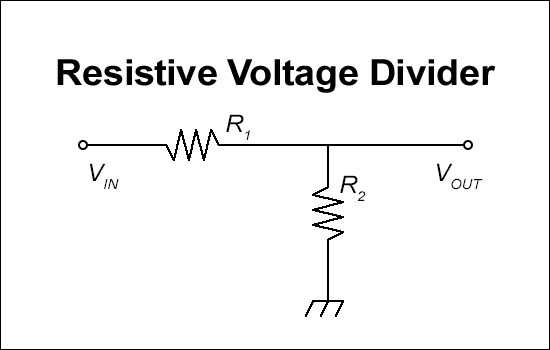# Output Voltage of Resistive Voltage Divider

This calculator calculates the output voltage of a resistive voltage divider.

Output values are rounded to the second decimal point.

Formula

Vout = (R2/(R1+R2)) * Vin

Where "Vout" is the output voltage, "R1"&"R2" is resistance of corresponding resistors, and "Vin" is the input voltage.### Input

R1:

R2:

Input Voltage: Volts